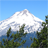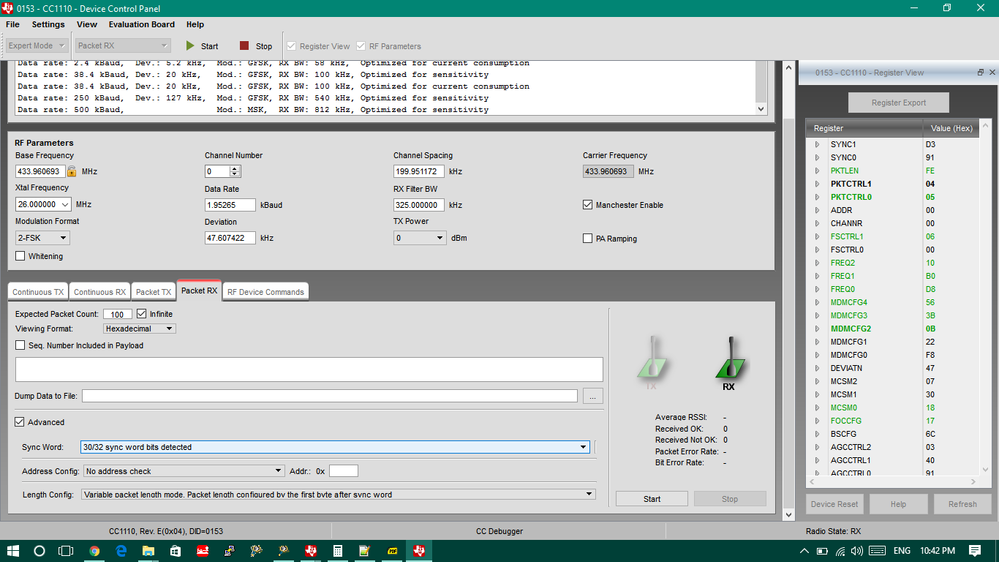## CC1110 as FXTH87 434Mhz Reciever

cancel
Showing results for
Did you mean:

## CC1110 as FXTH87 434Mhz Reciever

649 ViewsContributor I

Hello Everyone,

I am using fxth870911d to transmit sensor data at 434 Mhz,  I am initializing the fxth87 chip as follow,

``````void Init_RF(void) {
/* Turn module on in case it wasn't */
TPMS_RF_ENABLE (SET);

RFCR0 = 249; //2000 bps

RFCR1 = 0x78;    // set to 78 for 120 bits - largest frame

RFCR2 = 0x0E;    // RF Transmission OFF - No EOM - Pout=5dBm    - RPAGE=0
// 00001110

RFCR3 = 0x00;    // RF Output Low - RF Power UP - One Frame Transmitted
// 00000000

RFCR4 = 0x01;     //Interframe timing set to 1

RFCR5 = 0x00;     //No Pseudo-random number used

RFCR6 = 0x01;     //VCO highest Power - interframe timing
// was 00
RFCR7 = 0x08;     // RF Interrupt Enabled - LVD Disable - RFM Not reset

// fDATA0=fXTAL x ((12 + 4 x CF) + AFREQ/8192)
//       = 26MHz x((12 + 4 x 1) + 5644 (10110000 01100) /8192)
//       = 433.9131

// fDATA1=fXTAL x ((12 + 4 x CF) + BFREQ/8192)
//       = 26MHz x((12 + 4 x 1) + 5674 (1011000101010) /8192)
//       = 434.0083

// 434.0083 - 433.9131 = 47.6kHz

/* 434MHz */
PLLCR0=0xB0;// 1011 0000
//  AFREQ[12:5]

PLLCR1=0x60;//  01100      0   00
//  AFREQ[4:0] POL CODE[1:0]

PLLCR2=0xB1;// 10110001
PLLCR3=0x56;// 01010 110  CF=1 434MHz   MOD=1 FSK   CKREF=0 DX signal not generated

return;
}‍‍‍‍‍‍‍‍‍‍‍‍‍‍‍‍‍‍‍‍‍‍‍‍‍‍‍‍‍‍‍‍‍‍‍‍‍‍‍‍‍‍‍‍``````

Now I am transmitting the data with the following function.

``````void Fill_RFBUFFER(void) {

UINT8 au8RFDataForCS; // Maximum length is 32 bytes; size of the RF buffer (in bytes)
UINT16 Verification_Value;

/* Gather data into a local array that is to be loaded into the RF */

au8RFDataForCS[0u] = (UINT8) (0xAA);                          // Preamble
au8RFDataForCS[1u] = (UINT8) (0xAA);                          // Preamble
au8RFDataForCS[2u] = (UINT8) (0xAA);                          // Preamble
au8RFDataForCS[3u] = (UINT8) (0xAA);                          // //pREAMBLE
au8RFDataForCS[4u] = (UINT8) (0xD3);                          // Sync word
au8RFDataForCS[5u] = (UINT8) (0x91);                        // Sync word
au8RFDataForCS[6u] = (UINT8) (0xD3);                        // Sync word
au8RFDataForCS[7u] = (UINT8) (0x91);                        // Sync word
//au8RFDataForCS[9u] = (UINT8) (Tire_ID >> 24u);                // Tire ID
//au8RFDataForCS[10u] = (UINT8) (Tire_ID >> 16u);           // Tire ID
//au8RFDataForCS[11u] = (UINT8) (Tire_ID >> 8u);                   // Tire ID
//au8RFDataForCS[12u] = (UINT8) (Tire_ID);                      // Tire ID

au8RFDataForCS[9u] = (UINT8) (0x33);       // Tire ID
au8RFDataForCS[10u] = (UINT8) (0x34);         // Tire ID
au8RFDataForCS[11u] = (UINT8) (0x35);      // Tire ID
au8RFDataForCS[12u] = (UINT8) (0x36);      // Tire ID

au8RFDataForCS[13u] = (UINT8) (0x37);    // Firmware Version
au8RFDataForCS[14u] = (UINT8) (0x38);     // Derivative Descriptor
au8RFDataForCS[15u] = (UINT8) (0x39);
au8RFDataForCS[16u] = (UINT8) (0x40);
au8RFDataForCS[17u] = (UINT8) (u16CompAccelZ >> 8u);      // Z-axis acceleration
au8RFDataForCS[18u] = (UINT8) (u16CompAccelZ);
au8RFDataForCS[19u] = (UINT8) (u16CompAccelX >> 8u);      // X-axis acceleration
au8RFDataForCS[20u] = (UINT8) (u16CompAccelX);
au8RFDataForCS[21u] = (UINT8) (gu8CompVolt);                // Voltage
au8RFDataForCS[22u] = (UINT8) (gu8CompTemp);                // Temperature
au8RFDataForCS[24u] = (UINT8) (u8StatusAcqComp);           // Status Acquisition for COMP functions
au8RFDataForCS[25u] = (UINT8) (FrameID >> 8);               // Frame ID: keep alive counter
au8RFDataForCS[26u] = (UINT8) (FrameID);
au8RFDataForCS[27u] = (UINT8) (0xC0);                          // Fixed data => can be modified by the user
au8RFDataForCS[28u] = (UINT8) (0xC1);                          // Fixed data => can be modified by the user
au8RFDataForCS[29u] = (UINT8) (0xC2);                          // Fixed data => can be modified by the user

/* Calculate CRC and add it at the end of the frame */
Verification_Value = Calculate_CRC_MKW01( &au8RFDataForCS, (UINT8)(Payload_Length + 1));
au8RFDataForCS[30u] = (UINT8) (Verification_Value >> 8); // CRC
au8RFDataForCS[31u] = (UINT8) (Verification_Value);

TPMS_RF_WRITE_DATA (sizeof(au8RFDataForCS), &(au8RFDataForCS), 0u);
TPMS_RF_SET_TX(255);
}‍‍‍‍‍‍‍‍‍‍‍‍‍‍‍‍‍‍‍‍‍‍‍‍‍‍‍‍‍‍‍‍‍‍‍‍‍‍‍‍‍‍‍‍‍‍‍‍‍‍‍‍‍‍‍‍‍‍``````

So, the summary is that Device is transmitting data with 2-FSK, Manchester coding, 4-preamble byte followed by 4 bytes of the sync, with 433.960693 Mhz as carrier frequency with a Frequency deviation of 47.607422 kHz, and the data rate is 2000bps.

Now, at the receiver end I am using, CC1110EMK + CC Debugger + SmartRF Studio, I have made the following setting at the receiver as shown in the screenshot below:I am not receiving any data at the receiver, Can anyone please guide me or give some hint, to resolve this problem.

Your any kind of support is appreciated, Thanks

Tags (3)
169 ViewsNXP Employee

Hello Sumir,

I hope my response does not come too late.

I do not see any problem with the device initialization.

Regarding the Fill_RFBUFFER() function, I noticed that you modified the example code, have you tried to use the default values? I mean, the values shown in the example code. Can you please share your results?

The Preamble and sync word need to be configured the same in both the receiver and the TPMS transmitter. Are these parameters configured as mentioned in the receiver?

Unfortunately, I am not familiar with the receiver mentioned. In this case, I would like to recommend using the Using the Kinetis KW01 as the Receiver.